Acute, Obtuse, Reflex Angles

Chapter 5 Class 6 Understanding Elementary Shapes
Concept wise

We studied that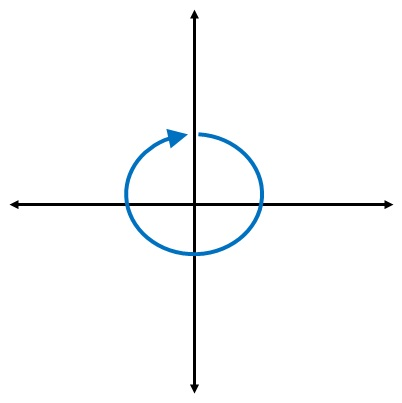1 revolution = Full angle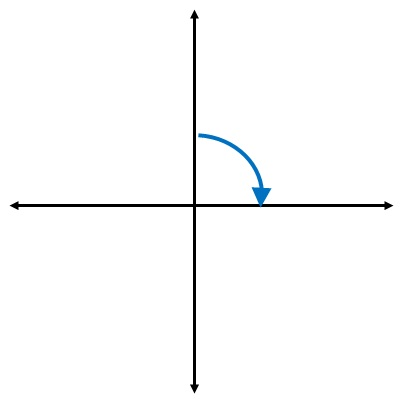1/4 revolution = Right Angle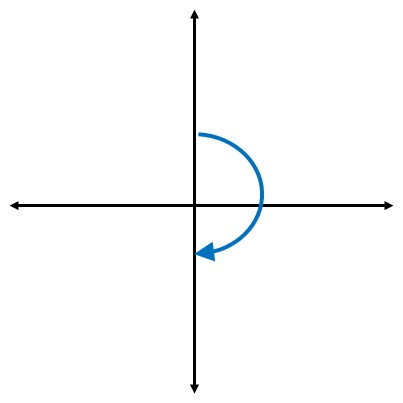1/2 revolution = Straight angle

Now,

Acute angle is less than right angle.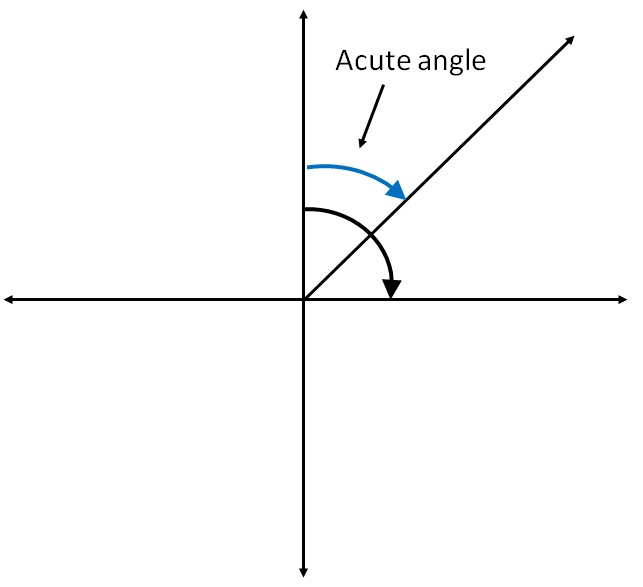Other examples are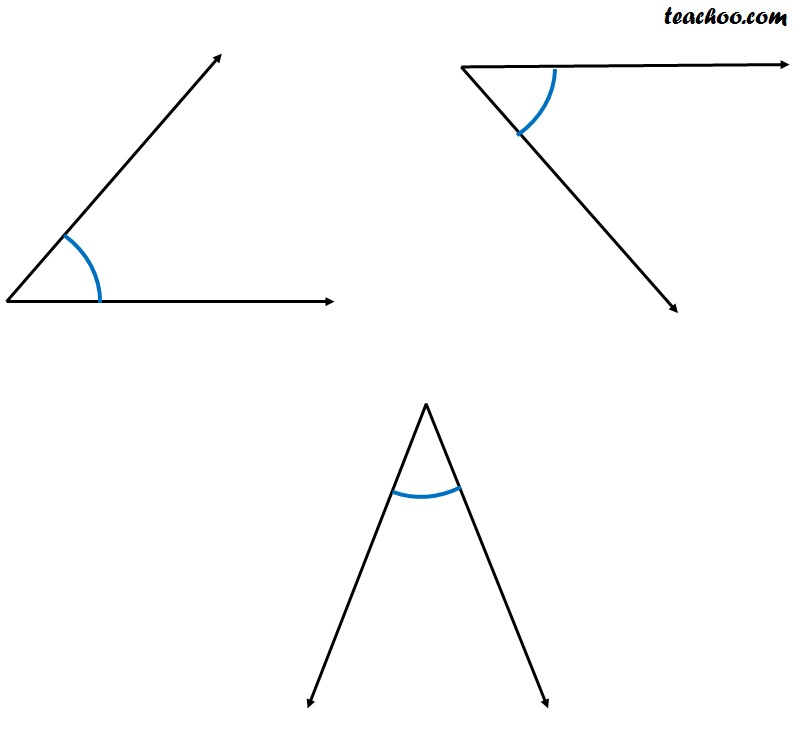#### Obtuse Angles

Obtuse angle is angle greater than a right angle but smaller than a straight angle.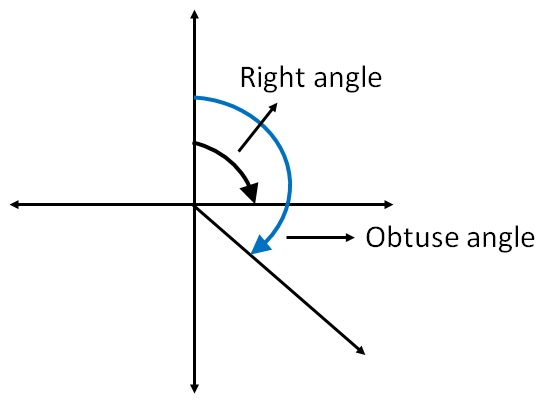Other examples are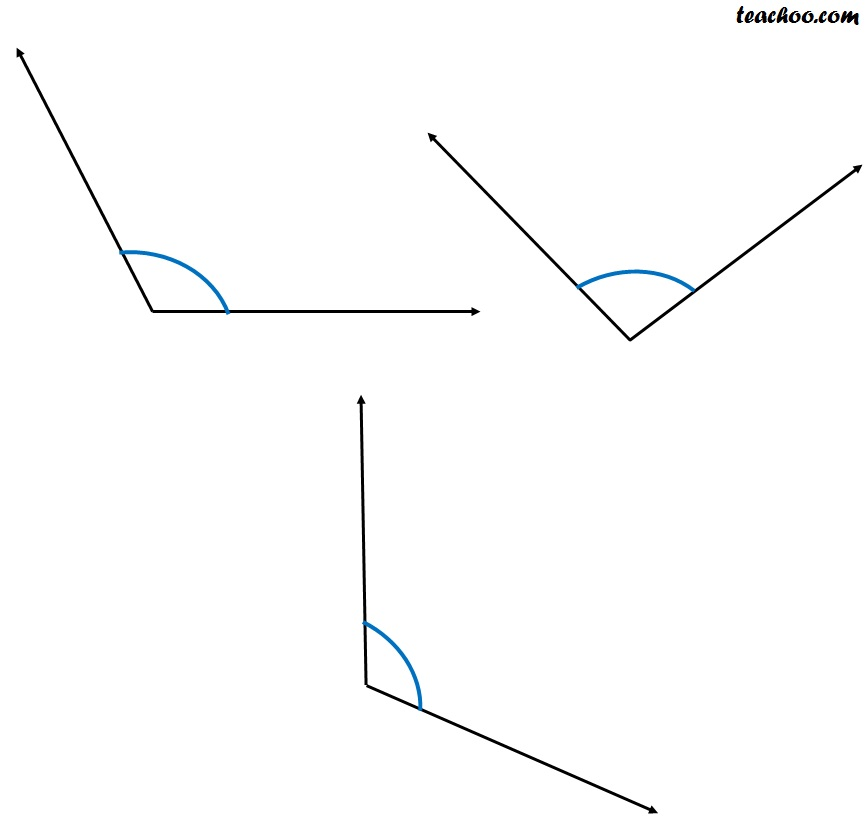#### Reflex Angles

Reflex angle is greater than a straight angle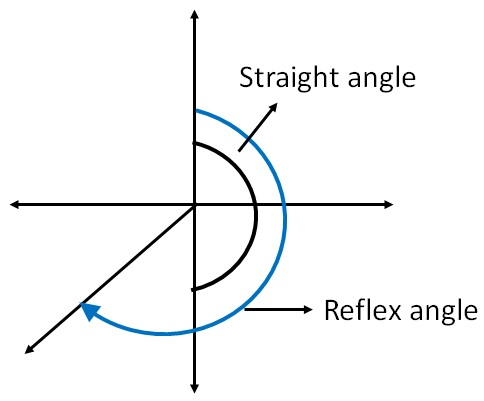Other examples are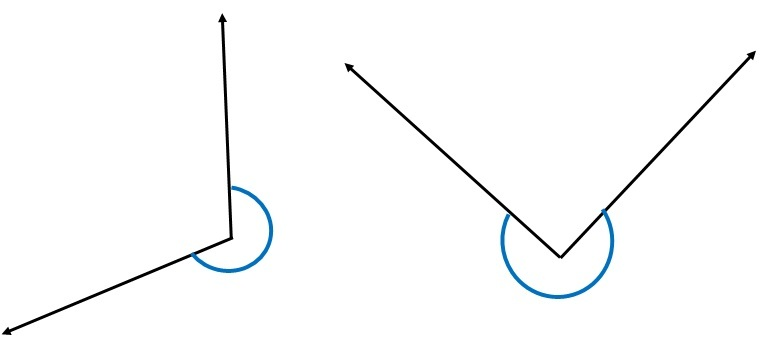Learn in your speed, with individual attention - Teachoo Maths 1-on-1 Class